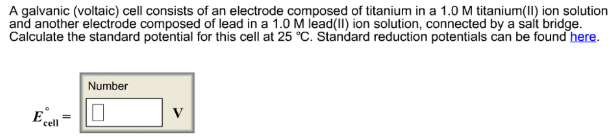# Problem: A galvanic (voltaic) cell consists of an electrode composed of titanium in a 1.0 M titanium(ll) ion solution and another electrode composed of lead in a 1.0 M lead(ll) ion solution, connected by a salt bridge. Calculate the standard potential for this cell at 25°C. Standard reduction potentials can be found here. E°cell =

###### FREE Expert Solution
95% (228 ratings)###### Problem Details

A galvanic (voltaic) cell consists of an electrode composed of titanium in a 1.0 M titanium(ll) ion solution and another electrode composed of lead in a 1.0 M lead(ll) ion solution, connected by a salt bridge. Calculate the standard potential for this cell at 25°C. Standard reduction potentials can be found here.

cell =